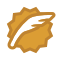# 程序员的数学--用贝叶斯定理来推断一个案子

1. 先给一个假设
2. 然后观察验证和修改
3. 得到相对靠谱的结果

1. 假设他是正人君子的概率是 0.5
2. 寻求证据，证明他是正人君子或者否
3. 修正之前的假设概率得到最终概率

### 什么是贝叶斯定理？

P ( H ∣ E ) = P ( H ) ∗ P ( E ∣ H ) / P ( E ) P(H|E)=P(H)*P(E|H)/P(E)

### 条件概率

P(E|H) 是条件概率，当事件 H 发生时，事件 E 也发生的概率是多少

#### 标准似然度 1

P ( E ∣ H ) / P ( E ) P(E|H)/P(E)

### 后验概率

P(H|E) 是条件概率，但因为标准似然度的原因，它被称为后验概率。
P ( 后 验 ) = 标 准 似 然 度 ∗ P （ 先 验 ) P(后验)=标准似然度*P（先验)

### 贝叶斯推理尝试

#### 1. 给假设概率

P ( H ) = 0.6 P(H) = 0.6

#### 2. 观察论证

1. 我一直以为你是正人君子，直到发现你最终经常做一些猥琐的动作。
2. 我一直以为你是正人君子，直到发现你每天读《曾国潘家书》。

P ( E ) = P ( E ∣ H ) ∗ P ( H ) + P ( E ∣ A ) ∗ P （ A ） P(E) = P(E|H)*P(H) + P(E|A)*P（A）

P(E) 的意义是一个人做猥琐动作的概率，包括了正人君子做猥琐动作的概率和猥琐男做猥琐动作的概率。

P ( E ∣ H ) = 5 / 60 = 0.083 P(E|H)=5/60=0.083

P ( E ∣ A ) = 36 / 40 = 0.9 P(E|A)=36/40=0.9

P ( E ) = P ( E ∣ H ) ∗ P ( H ) + P ( E ∣ A ) ∗ P （ A ） = 0.083 ∗ 0.6 + 0.9 ∗ 0.4 = 0.05 + 0.36 = 0.41 P(E) = P(E|H)*P(H) + P(E|A)*P（A）=0.083*0.6+0.9*0.4=0.05+0.36=0.41

P ( H ∣ E ) = P ( H ) ∗ P ( E ∣ H ) / P ( E ) = 0.6 ∗ 0.083 / 0.41 = 0.12 P(H|E)=P(H)*P(E|H)/P(E)=0.6*0.083/0.41=0.12

### 数学题目练习

A 代表男孩子，所以 P(A) 为 0.45

B 代表女孩子，所以P(B) 为 0.55

E 代表扔东西，P(E|A) = 0.6,P(E|B)=0.4，所以：
P ( E ) = P ( E ∣ A ) ∗ P ( A ) + P ( E ∣ B ) ∗ P ( B ) = 0.6 ∗ 0.45 + 0.4 ∗ 0.55 = 0.49 P(E)=P(E|A)*P(A)+P(E|B)*P(B)=0.6*0.45+0.4*0.55= 0.49

P ( A ∣ E ) = P ( A ) ∗ P ( E ∣ A ) / P ( E ) = 0.45 ∗ 0.6 / 0.49 = 0.55 P(A|E)=P(A)*P(E|A)/P(E)=0.45*0.6/0.49=0.55

### 贝叶斯定理的影响因子

P ( H ∣ E ) = P ( H ) ∗ P ( E ∣ H ) / P ( E ) P(H|E)=P(H)*P(E|H)/P(E)

P ( E ) = P ( E ∣ H ) ∗ P ( H ) + P ( E ∣ A ) ∗ P （ A ） P(E) = P(E|H)*P(H) + P(E|A)*P（A）

1. P(H)
2. P(A)
3. P(E|H)
4. P(E|A)

### 参考

1. 王丽. 浅析贝叶斯公式及其在概率推理中的应用[J]. 科技创新导报,2010,(24):136 ↩︎

2. Alex Edmans.What to trust in a post-truth world.https://www.ted.com ↩︎

frank909CSDN认证博客专家 CV(computer vision)

01-071771
09-2886710-033578
08-10670
04-091476
04-0822点击重新获取扫码支付余额充值